# An optimization example - Writing fast Commodore 64 Basic code

## Base versionLet's say we're just starting to write a game where the player controls a lawnmower. First, we need to print the lawn. We decide it should be ten rows high and 31 columns wide. Here's a first, reasonable implementation. To aid our optimization efforts, we start by resetting the real time clock, and at the end of the program we print the number of elapsed jiffies (1 second = 60 jiffies). With this initial version of the program, it takes 339 jiffies, which is the same as 5.65 seconds. Now, let's see if we can improve on that.

Note: We would normally want to remove all spaces in the code, but I have decided to keep them to make it easier to follow this example.

## Shorter variable namesFirst, we'll make all the variable names single character. This doesn't make a huge difference here, but it does shave of 6 jiffies, thus lowering the time by almost 2%.

## Fewer lines of code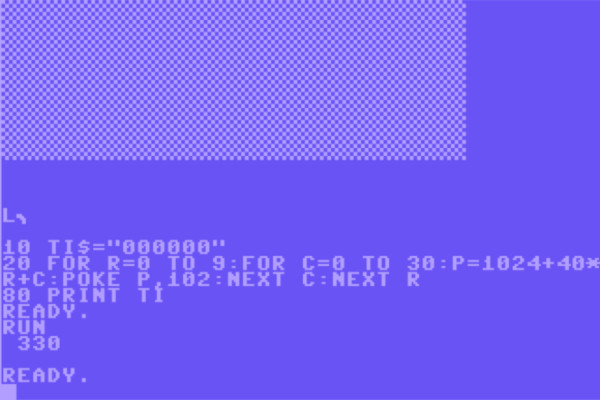Next, let's write the lawn printing code on a single line instead of six. This shaves off another 3 jiffies, or almost 1% of the time.

## Remove loop variables in NEXTWe don't actually need to tell Basic which loop a certain NEXT statement belongs to. By skipping the variable name in the NEXT, we make the parsing faster. Here, we lower the time by another six jiffies.

## Skip multiplication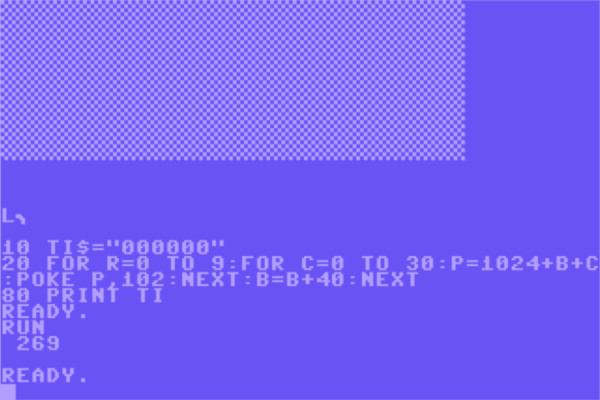The code inside the inner loop, i.e. the assignment of P and the POKE statement, is executed 10 * 31 = 310 times. And on each of these iterations, we perform a multiplication. This is expensive, and easily avoidable. Instead of multiplying the current row number (R) by 40 each time, we introduce a variable B, which starts at 0 and then we add 40 after each row. Instead of performing 310 multiplications, we now perform ten additions. This is way cheaper, so we save 55 jiffies, which is a saving of 17%.

## Don't store intermediate resultInside the inner loop, we're assigning a value to P, which we then use once. Storing the value in the variable takes time, and retrieving it takes time. We'll move the calculation to the POKE statement instead. This saves 27 jiffies, which is 10% of the time.

## Initialize variables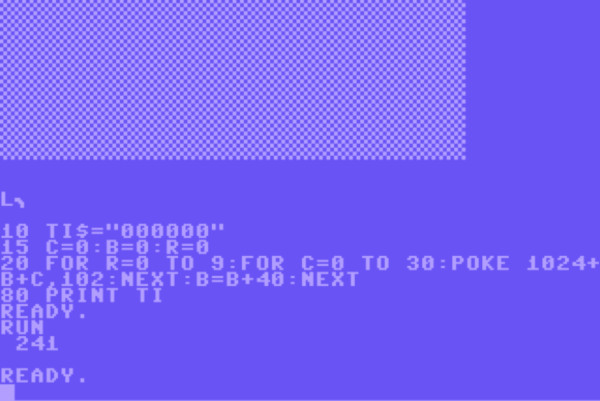Variables are stored in the order of first assignment. Since we want the variables that we use the most to be the fastest to access, let's assign values to the variables in order of importance. C is incremented 310 times and read 310 times, so we'll initalize it first. B is read 310 times and assigned to ten times, so B comes next. R is just incremented ten times, so R goes last. This change only saved us one jiffy. This is because we only have three variables, meaning that all of them can be accessd pretty quickly anyway. When our game is finished, we probably have a hundred variables or more, and this step has a much larger impact.

## Use . instead of 0It's very easy to use "." instead of 0, and it saves a little time. Since none of these code changes occur inside the inner loop, the impact is quite small - just one jiffy.

## Use variables to hold constants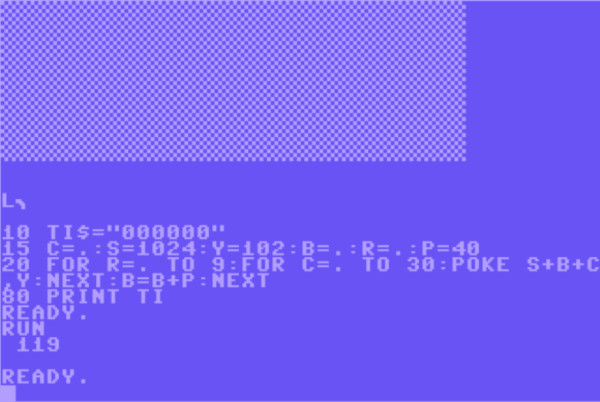Parsing a numeric constant and turning it into a floating point number is quite expensive, and the cost is relative to the number of digits. By putting 1024 into the variable S, 102 in variable Y and 40 in variable P, we manage to save 121 jiffies, which is 50% of the time used!

## Simplify calculation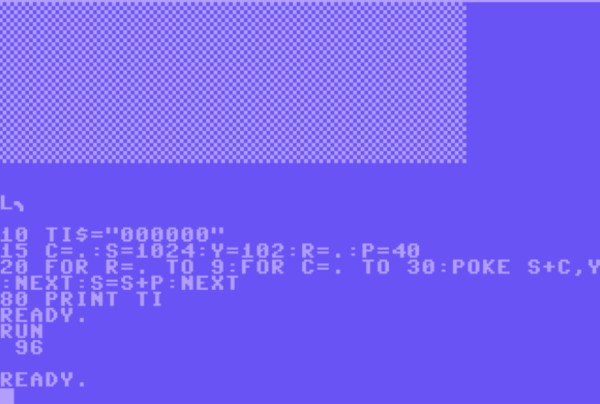When B is used, it's always added to S. So, we don't actually need these two as separate variables. Instead of adding 40 to B after each line, we add 40 to S. This means we can skip B in the calculation for the POKE statement, thus saving 23 jiffies, or 19% of the time used.

## Simplify calculation even more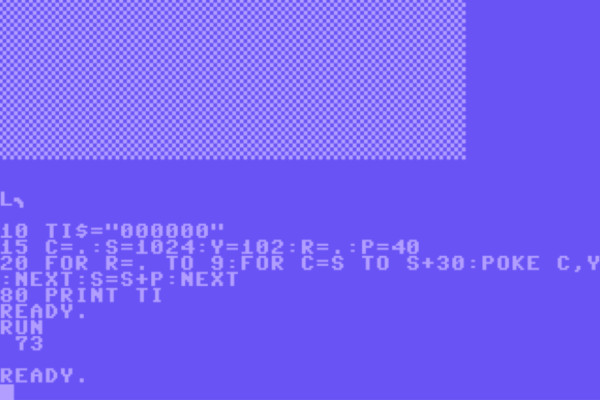Instead of adding S and C on each iteration of the inner loop, we can incorporate the value of S into C, in the FOR statement. This saves another 23 jiffies, or 24% of the time used. Compared to our first implementation, we have now reduced the execution time by 78.5%

## Use PRINT instead of POKE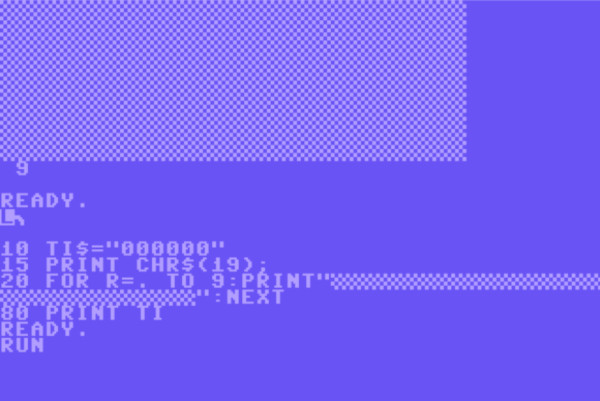Now suddenly, we realize that we can print the lawn using ten PRINT statements instead of 310 POKEs. Let's try that. We reduce the time from 73 jiffies to 9 (this is now printed just below the lawn), thus saving 88% of the time used. Compared to our very first implementation, we have decreased the execution time by 97.3%. Not bad at all.

## Conclusion

This was meant to show off a number of useful techniques in a realistic optimization scenario. As you can see, some of the techniques yielded huge savings in execution time, while others were not as important. However, all the little savings add up to a quite substantial saving as well. Also, the effect they have may be greater in a bigger program.

Fredrik Ramsberg, 2019. microheaven.com